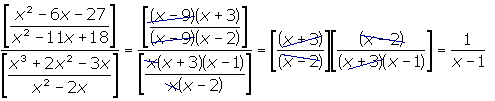SEARCH HOMEMath Central Quandaries & QueriesQuestion from a student: I have a question that continues to lead me to the answer x/(x - 1) but according to my math book, the answer should be 1/(x - 1). Who is right? Who is wrong? Here is the question: (x^2 - 6x - 27)/(x^2 - 11x + 18) DIVIDED by (x^3 + 2x^2 - 3x)/(x^2 - 2x)Hi there.

First, I factored the polynomials. Then I multiplied by the reciprocal of the denominator instead of dividing by the fraction. The book is right.Hope this helps,
Stephen La Rocque.Math Central is supported by the University of Regina and The Pacific Institute for the Mathematical Sciences.